# Amazing Maze Maker in MakeCode for Minecraft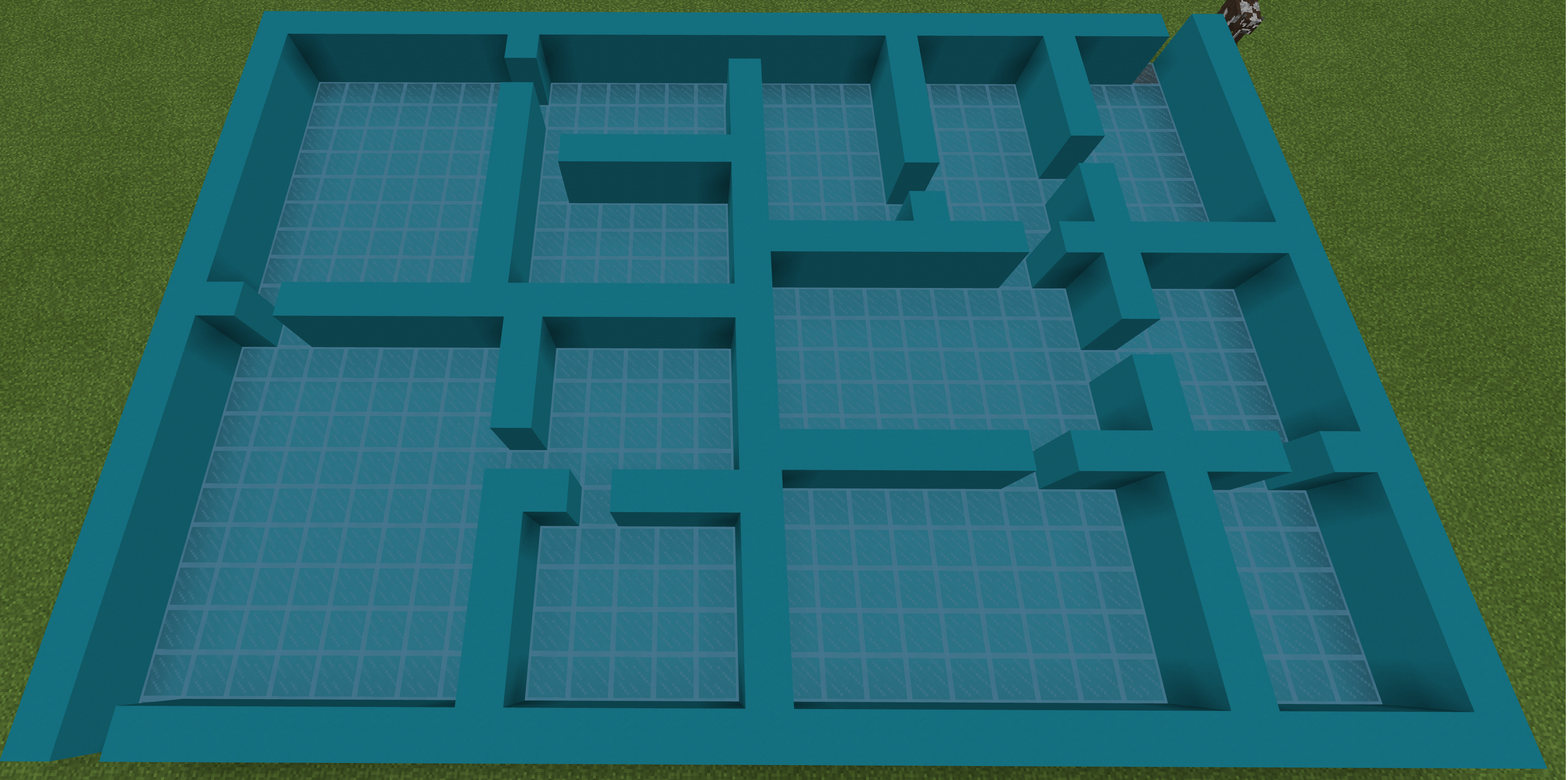The mazes generated by Amazing Maze Maker can be used by Mazer, the Maze Walking Snake in MakeCode for Minecraft.

## Using Amazing Maze Maker

### Run Chat Command Generates a Single Maze

The run chat command with no arguments generates the default maze, which is  10 blocks x 10 blocks with minimum wall of 4 blocks.

The run chat command can have the following three arguments:

• num1 — the number of blocks for the X direction (east/west). Minimum 10.
• num2 — the number of blocks for the Z direction (north/south). Minimum 10. If Z is 0, it is set to num1 generating a square maze.
• num3 — the minimum number of blocks in a wall. Must be 4 or greater. Default is 4. If needed, num1 or num2 are adjusted so that each side can be divided resulting in wo areas that are larger than num3 (the minimum).

### Maze Colors Can be Changed in On Start Block

The blocks from which a maze is built can be changed in the on-start block shown below. If using the program Mazer to walk the maze, the 3 maze blocks must also be changed in Mazer to match the ones here.### Erase Chat Command Erases the Maze

If the player stands  on the ground left of the sunflower and a few blocks west so that the maze is in front, this command will erase a maze so that the area can be used to generate another. Instead of erasing, however, a player can just move to another part of the world to generate another maze.

## The Algorithm

Amazing Maze Maker employs the recursive-division maze generation algorithm. This algorithm creates a maze with no loops; that is, a simply connected maze, which is also called a perfect maze.

The algorithm is called “recursive division” becuase it uses recursion to build the maze. Recursion in computer science is is a method of solving a problem where the solution depends on solutions to smaller instances of the same problem. In coding, this means that a function calls itself or calls a function that calls the first function.

The drawing below is the pseudocode for the recursive-division maze generation algorithm. Pseudocode is a notation resembling a simplified programming language, used in program design. This drawing illustrates recursion because the function Divide calls the function Divide_NS, which calls the function Divide. The arrows indicate calls. Notice that the function Divide returns (exits) to its caller when a wall is so small it can no longer be divided into two walls that are larger than the minimum.## Coding Strategy

Recursion using MakeCoode blocks is challenging becuase MakeCode block functions cannot have parameters. This is not the place to rail against this MakeCode shortcomings, but believe me, this particular one keeps me frustrated. Yes, I know that I could have used JavaScript,, but I prefer to stay with those colorful, readable, loveable blocks.

The pseudocode above shows that the recursive function Divide takes three arguments: a position, a X length and a Z length. These three parameters define a rectangle as shown in the drawing below. In each area of the maze the X and Z arguments become smaller with each recursive call to divide until eventallly they asre too small to further divide and the calls unwind. When one area of the maze has been completely divided, the algorithm moves to another area and divides it recursively. This results because the function Divide_NS and Divide_EW each call Divide twice — once for the leff or bottom area of the current area and once for the right or top area of the current area.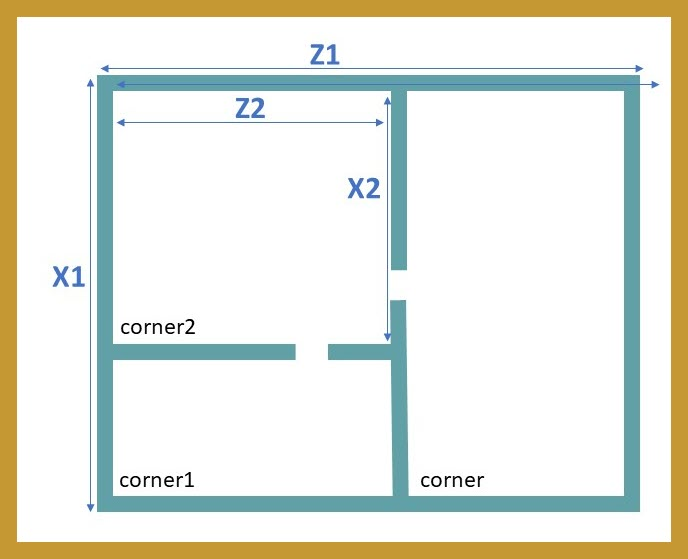Now, what to do about the fact that MakeCode function blocks do not allow parameters? I used three stacks (corner stack, X stack and Z stack) instead of parameters. Before each call to Divide, corner, X and Z values (that would have been arguments) are pushed onto the stack. Just before divide exits, the same three values are popped off the stack.

A stack is an abstract data type that operates as a LIFO (last in first out) list. At a minimum a stack has push and pop operations defined. Push puts an item on top of the stack and pop takes the top item off the stack. An optional peek operation enables viewing the top item on the stack without modifying the stack.

A stack may be implemented in a variety of ways. For this program, the push operation is implemented by adding an item to the front of a MakeCode list. The pop stack operation is implemented by removing a value from the front of the list. A stack could have equally well been implemented using the end of a list instead of the beginning, in which case, push would put a value at the end of the list and pop would take it off of the end.

## The Code

All the code is shown in the screenshot below.  In the screenshot to the left is the initialization code. The third column starts the maze. Columns 4 and 5 divide an area and create either a NS or an EW wall. Each section of the code will be explained in later sections of this post.### Run Starts the Program

Run calls several functions to check argument values, initialize stacks and set anchors. These three functions are defined towards the end of this post. The function do_maze launches the algorithm.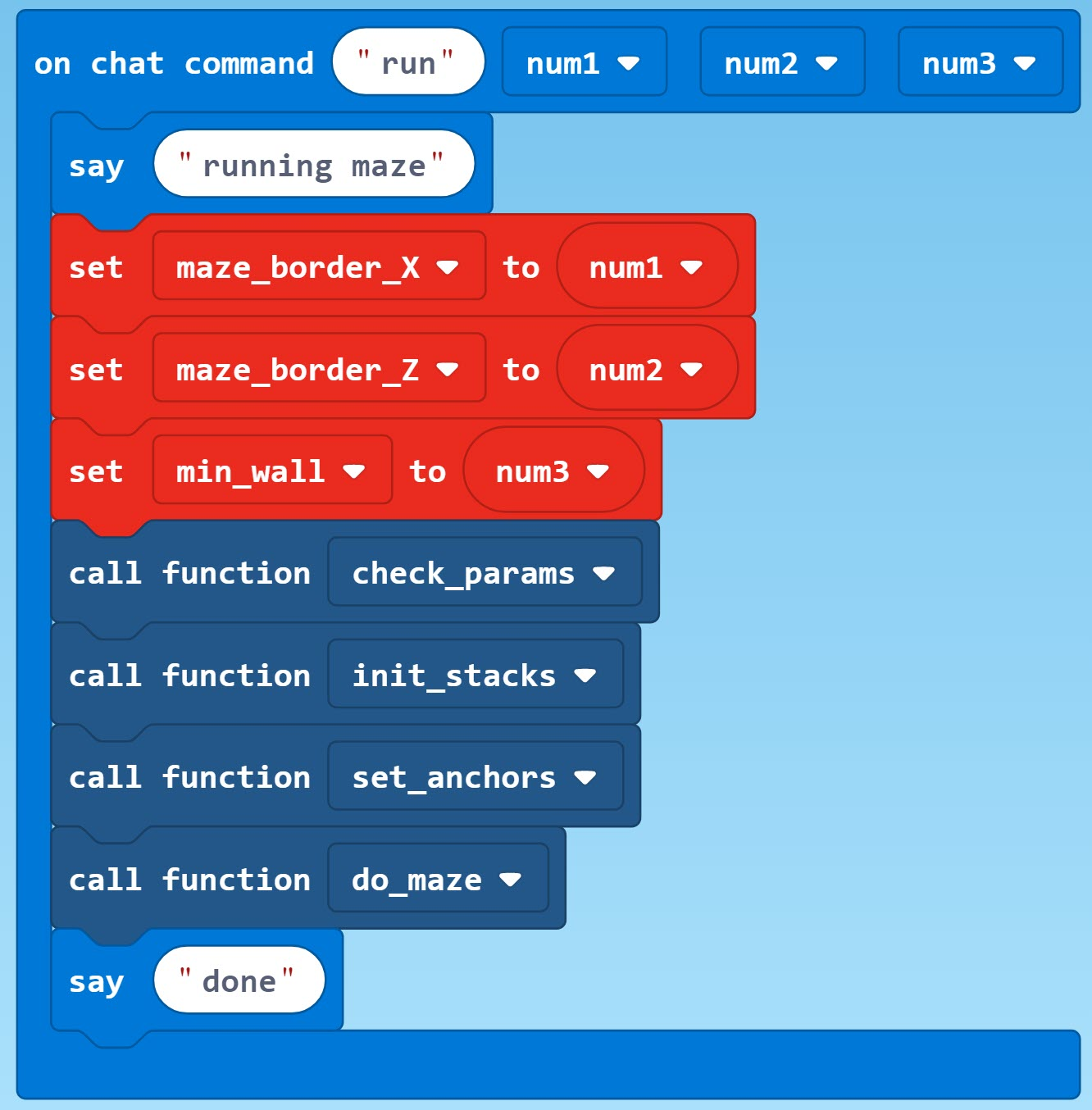### do_maze Calls Divide with Three Arguments Using Stacks

Notice that do_maze, pushes the required three arguments onto their respective stacks before calling divide.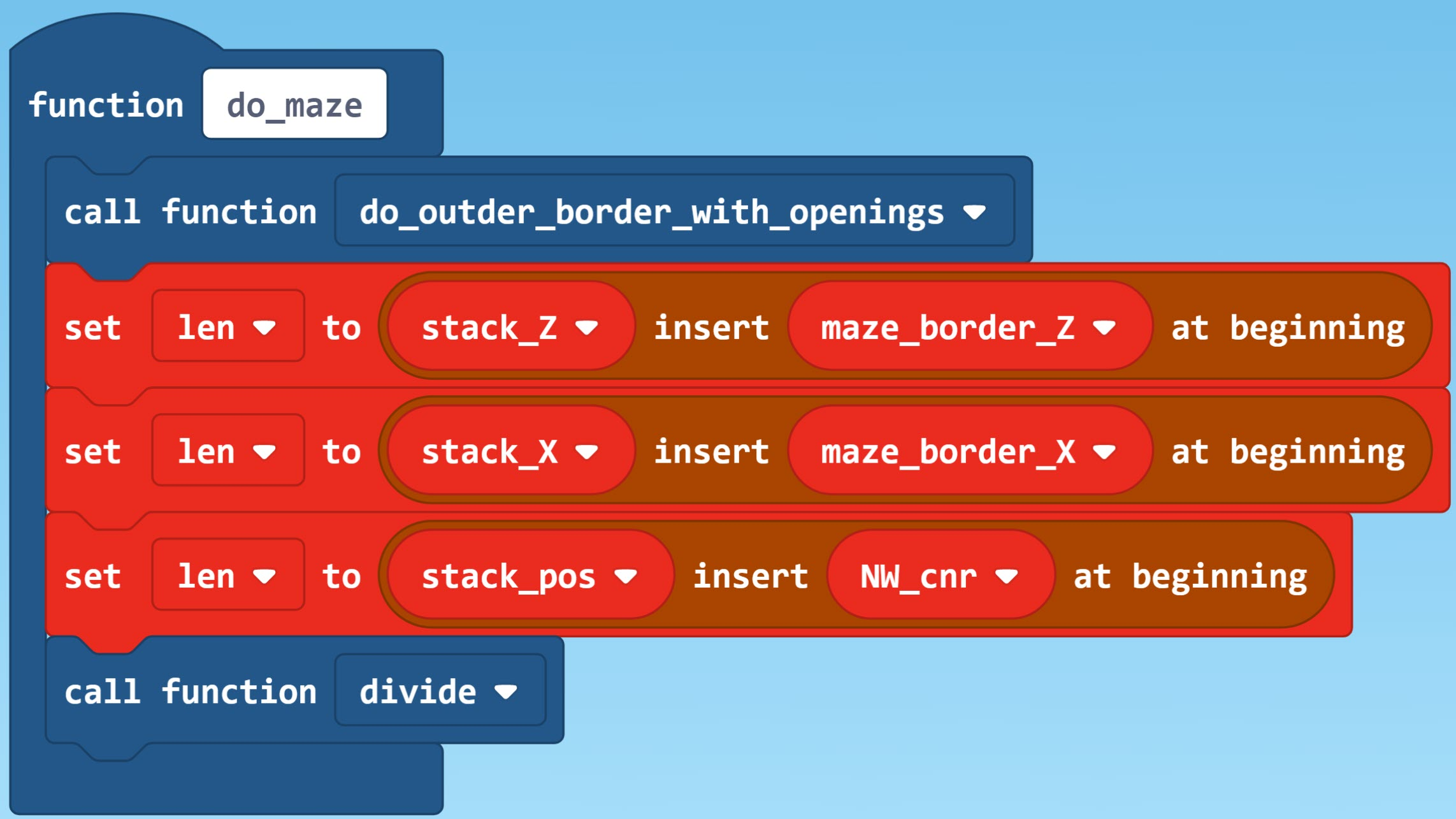### Divide Function Is Recursive

Depending on which wall (NS or EW) of the current area is longer, Divide calls either divide_along_NS_at_X or divide_along_EW_at_Z. If the longest wall is too short to divide into two at least  minimum length walls, divide exits, returning to the block after the block that called it.### Divide an Area and Make an Opening in the Dividing Wall

The two functions and their supporting routines are shown after this screenshot, which shows a potential problem in the placement of a wall. A wall dividing an area is placed randomly such that each resulting wall is longer than the minimum. The random position chosen might be such that one or both ends is at an opening in a previously placed wall. In the screensot below, the right horizontal wall blocks an opening in the vertical wall on its left. In the code to place a new wall (below), the functions get_new_wall_X and get_new_wall_Z search for an a wall that does not block an opening using the  functions try_wall_X and try_wall_Z.Notice that divide_along_NS_X below, calls divide twice — once for each area resulting from the wall it placed. The first thing that this function does is to get the three arguments from the three stacks. Conceptually, a peek operation is used to do this since the MakeCode list operation used dos not remove the value from the list. Careful examination of the code will reveal how the three  arguments are set up for each of the two calls to divide. At the end of the routine, he last function called pops each of the three stacks.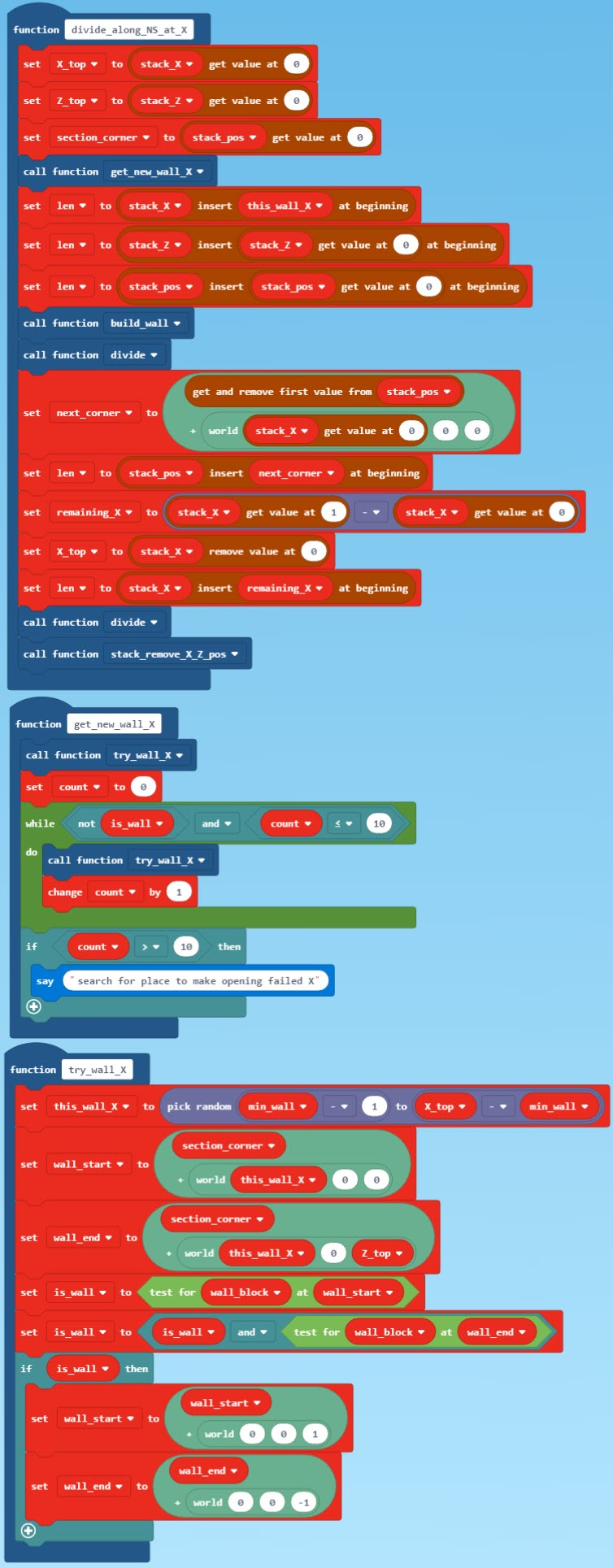The functions below divide_along_EW_at_Z differs rom the one above by the direction that the wall is placed.### Build Wall Function

Both the above functions call the function build_wall to place the wall between the endpoints calculated by the calling function. It also places an opening in the wall at a random location between the endpoints.### Initialize Stacks and Pop Top Items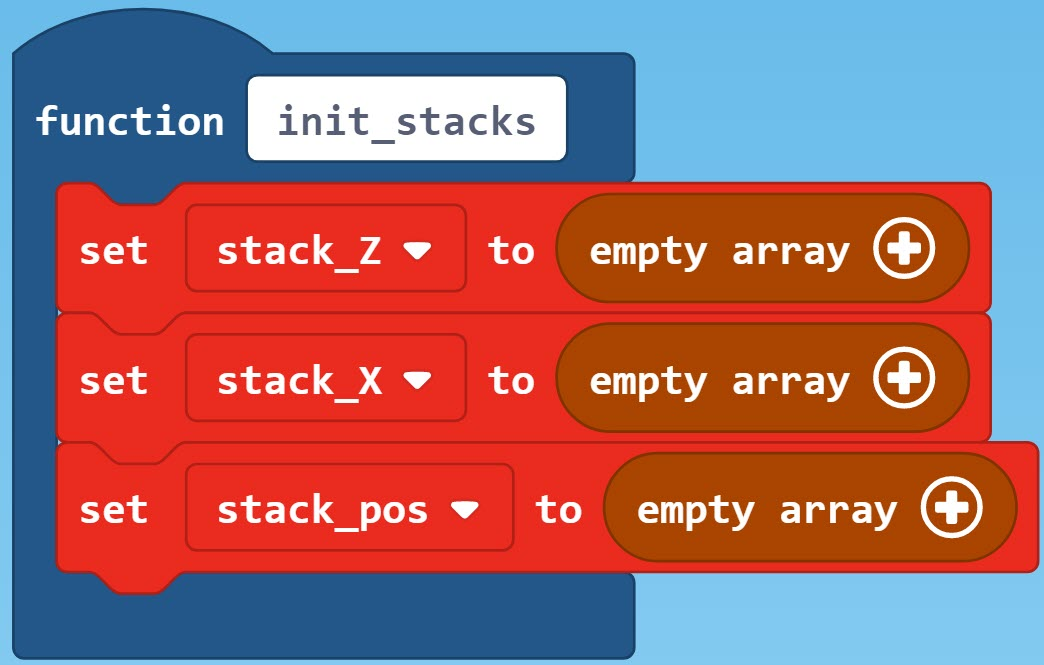The function below pops (removes the top) values from the three stacks that are arguments to Divide and its subordinate functions.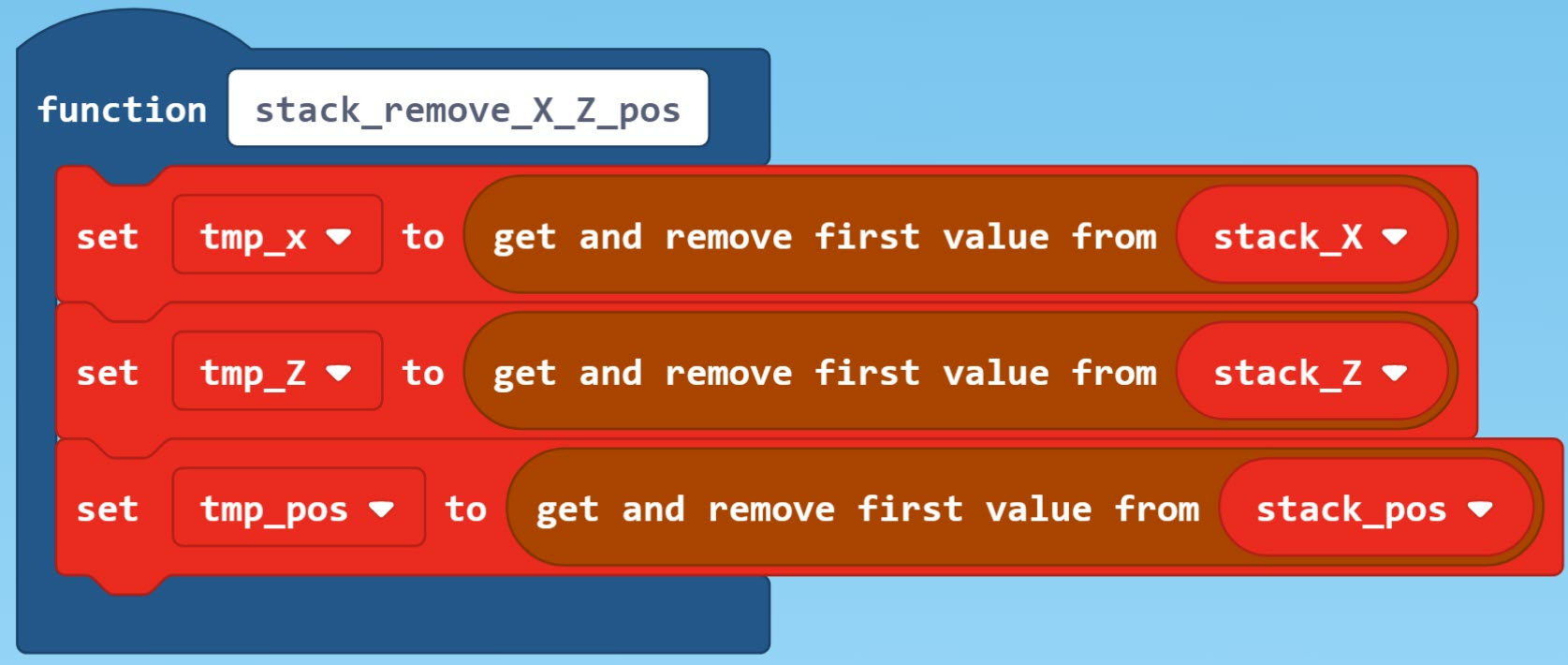### Check Parameters of the Run Chat Command

The function below checks the arguments proved to the run chat command.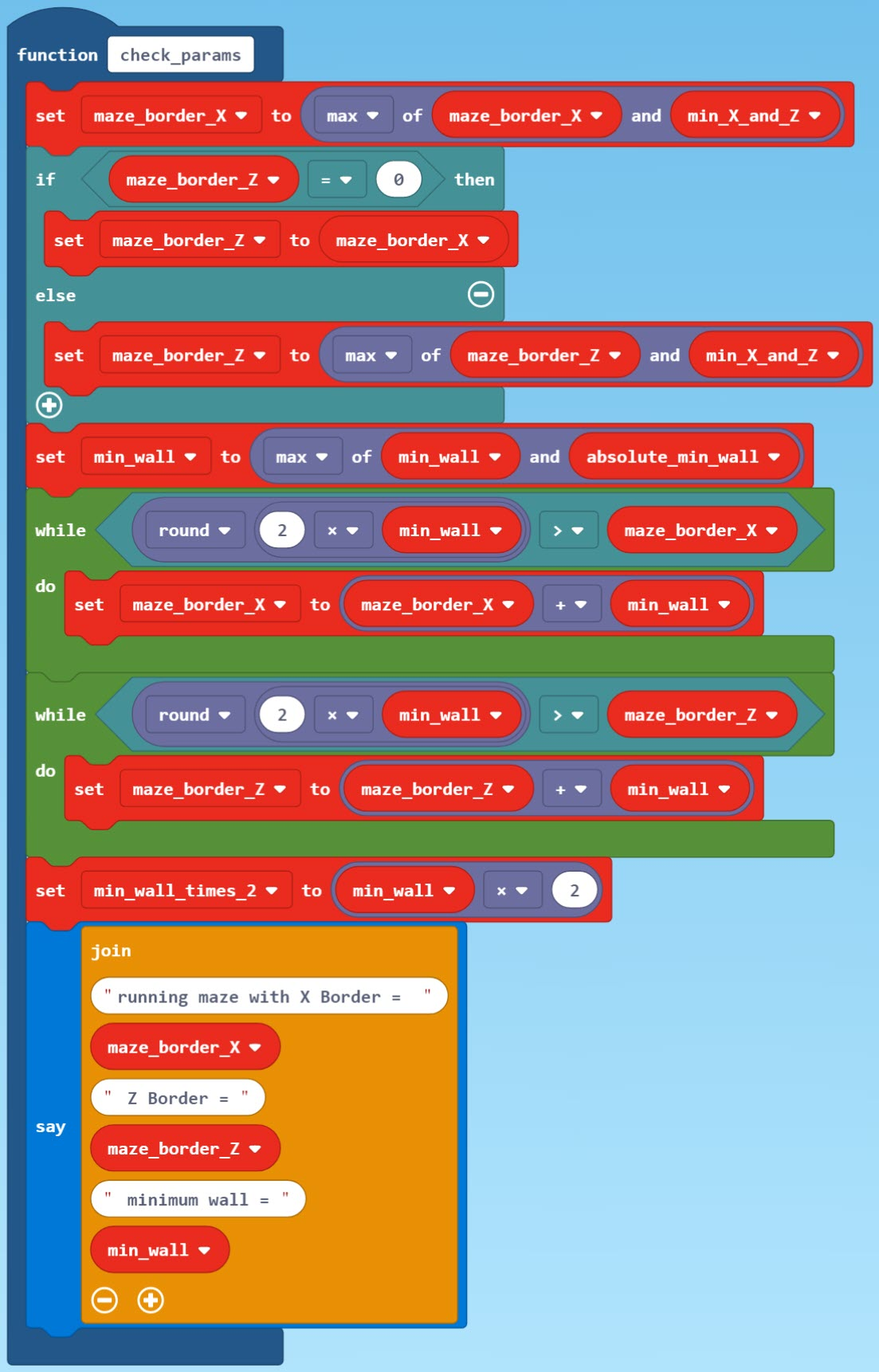### Set Anchors and Position Player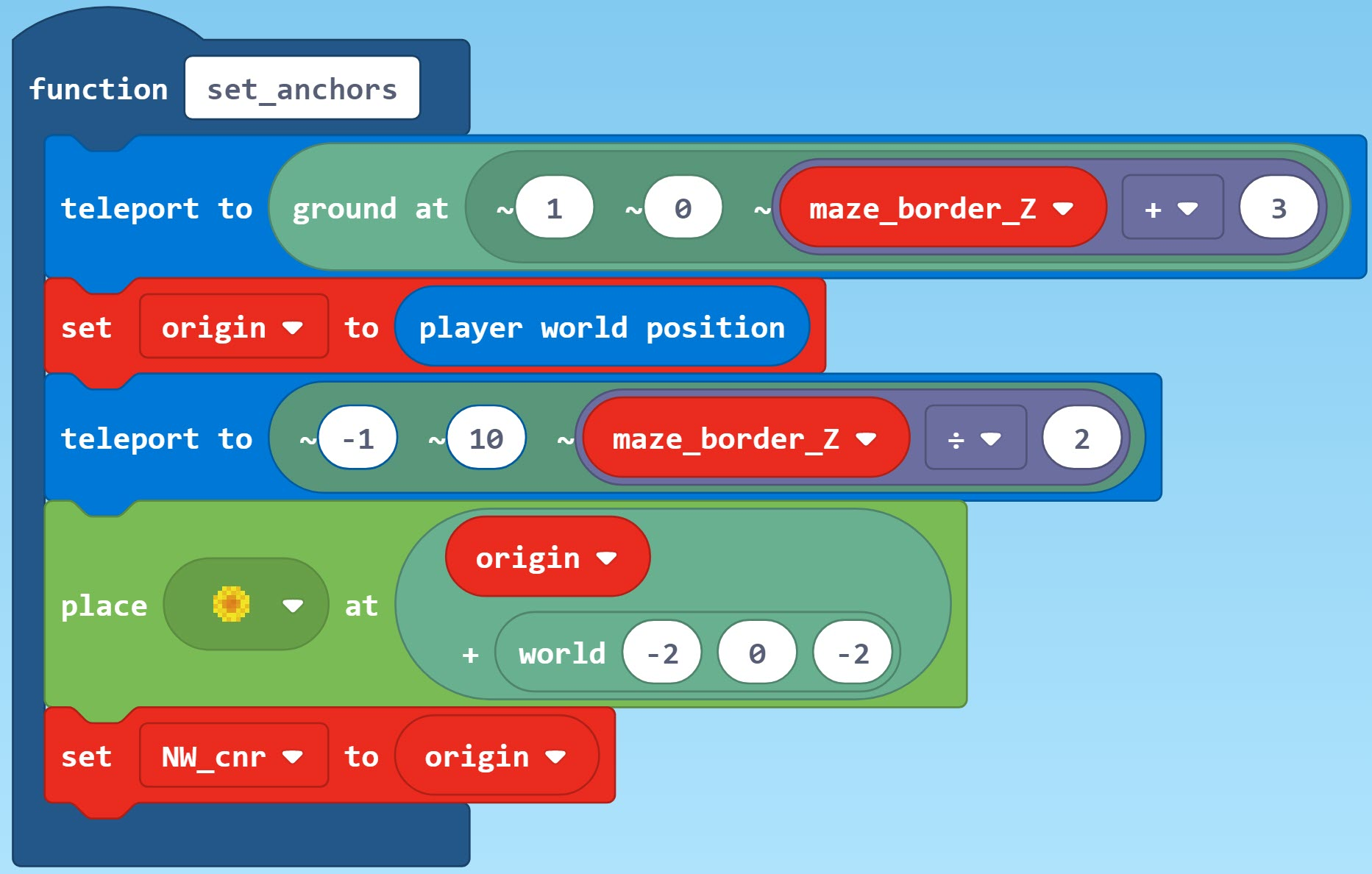### Function to Do Outer Maze Border with Openings### Erase Chat Command### Code Requires Flat World

This code assumes a flat world such as that described in MakeCode for Minecraft Sandbox World: Make It Flat and Simple.

## Get the Code

Amazing Maze Maker code is shared on Code Connection at this URL:

To get and use the code, follow these steps.

Click the Import button, which is upper right in the Code Connection window just below the banner. This will open the window shown below.Click the Import URL button, which is on the right, to open the window shown below.Paste the URL supplied for the program you want to use in the space under the text “Copy the URL …”Click the Go ahead! button.

The next window you will see will be the MakeCode window with the code downloaded from the URL. At this point, you can treat it like any other code, e.g., run it, save it locally, modify it, publish your changes or whatever else your heat desires.

We have tested several other methods of downloading the code using the URL, for example, pasting the URL in a browser. No joy. For more detailed instruction see our post How to Use Shared MakeCode on Microsoft Code Connection for Minecraft.